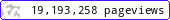•••••MadAsMaths.com :: Maths Booklets :: Standard Topics :: Integration

STANDARD TOPICS - INTEGRATION

These booklets are suitable for

• the second year Integral Calculus material, of a two year course in A Level mathematics.

• revision of Integral Calculus for undergraduate students in degrees with a significant amount of mathematics.

 integration_areas.pdfintegration_basics.pdfintegration_basics_student_version.pdfintegration_by_a_special_manipulation.pdfintegration_by_a_special_manipulation_student_version.pdfintegration_by_parts.pdfintegration_by_parts_student_version.pdfintegration_by_reverse_chain_rule.pdfintegration_by_reverse_chain_rule_student_version.pdfintegration_by_substitution.pdfintegration_by_substitution_student_version.pdfintegration_by_trigonometric_identities.pdfintegration_by_trigonometric_identities_student_version.pdfintegration_partial_fractions.pdfintegration_partial_fractions_student_version.pdfintegration_structured_exam_questions_part_i.pdfintegration_indefinite_mix.pdfintegration_indefinite_mix_student_version.pdfintegration_indefinite_mix_student_version_condense.pdfintegration_volume_of_revolution.pdfodes_context_modelling.pdfodes_separable_no_context.pdfparametric_integration_exam_questions.pdfintegration_numerical.pdf